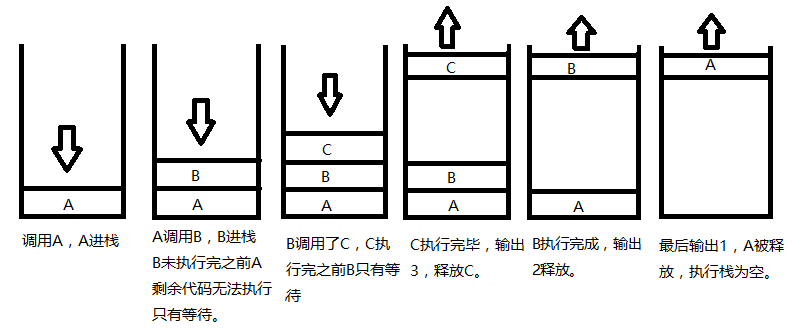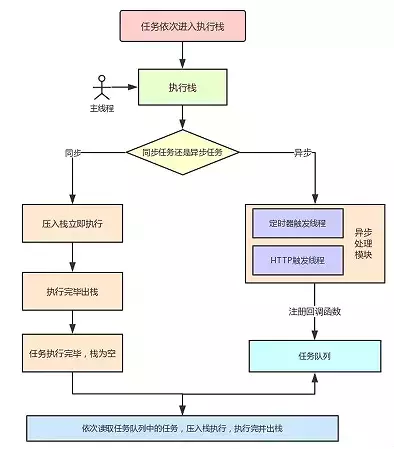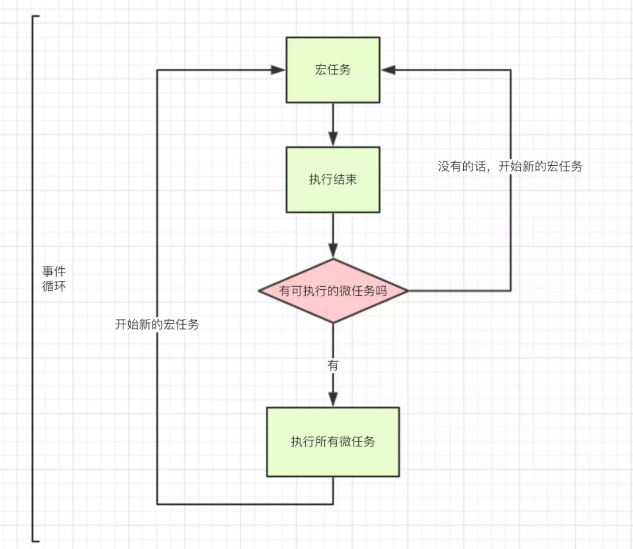# 听风是风

• 随笔 - 174
• 文章 - 0
• 评论 - 311
• 引用 - 0

## JS执行机制详解，定时器时间间隔的真正含义

壹 ❀ 引

let p = Promise.resolve(1);
p.then(resp => console.log(resp));
console.log(2);
//2
//1

let p1 = Promise.resolve(1);
p1.then(resp => console.log(resp));
let p2 = Promise.resolve(2);
p2.then(resp => console.log(resp));
//1
//2

setTimeout(() => console.log(2),0);
let p1 = Promise.resolve(1);
p1.then(resp => console.log(resp));
//1
//2贰 ❀ JavaScript中的同步异步

JavaScript是一门单线程非阻塞语言，在同一时间只能专心做一件事，如果前面的事情没做，后面的事情就得耐心的等着，这就是所谓的同步。

JavaScript本身是一门浏览器脚本语言，更多负责用户的交互，dom操作之类；假设JS并非单线程，我让两个行为同时操作一个dom对象，那岂不是乱套了。想想我们排队取餐吃饭，如果不排队，往往容易引发争吵，编程也是现实行为的抽象。

let funA = () => {
let NUM = 10000;
while (NUM) {
NUM--;
};
console.log(1);
};

let funB = () => console.log(2);

funA(); //1
funB(); //2叁 ❀ 执行栈与任务队列

let A = () => {
B()
console.log(1);
};
let B = () => {
C()
console.log(2);
};
let C = () => {
console.log(3);
};
A();//3 2 1肆 ❀ 事件循环 (Event Loop)伍 ❀ 宏任务与微任务

setTimeout(() => console.log('我第一'), 1000);
let p1 = Promise.resolve('我第二');
p1.then(resp => console.log(resp));
//我第二
//我第一

setTimeout(() => console.log('我第一'), 2000);
let p1 = Promise.resolve('我第二');
p1.then(resp => console.log(resp));
setTimeout(() => console.log('我第三'), 1000);
let p2 = Promise.resolve('我第四');
p2.then(resp => console.log(resp));
//我第二
//我第四
//我第三
//我第一图片来源

陆 ❀ 有趣的定时器

setTimeout(() => console.log('我第一'), 3000);

setTimeout(() => console.log('我第一'), 3000);
setTimeout(() => console.log('我第二'), 3000);

柒 ❀ 参考

posted on 2019-05-02 21:09  听风是风  阅读(...)  评论(...编辑  收藏